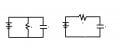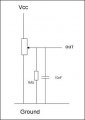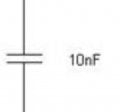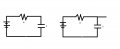# Resistors and Capacitors in series and parallel

#### KansaiRobot

Joined Jan 15, 2010
324
Hello everybody and thanks for all the help
How do you calculate a equivalent circuit or voltage when resistors and capacitors are put in series or parallel. All books have this dealing only with resistors or only with capacitors, but how about the mix??I ask this because for example in the following circuit if Vcc =5V and the Potentiometer is 10K. And let's suppose the wiper is in the middle (voltage divider of 5K 5K) how can I calculate the voltage at OUT???I usually make mistakes calculatingbut at least I know the theory that the resistance in the potentiometer (the 5K) and the other resistance 1M are in parallel so they can be replaced by only one resistance. But then what do I do with the capacitor??!

If someone can teach me this I will be greatly appreciated.

Kansai

#### ScottWang

Joined Aug 23, 2012
7,244
Assuming that the 1ok pot are separated by two fixed 5K as R1, R2 then the R2 5K which connected to ground can be in parallel with 1M to combines to a new resistor, in this situation that I call the R2 5K is the master, because its values is more less, and the 1M is the slave, because its values is more higher, almost you can ignore it, so you can calculates as :
Rn% = 1M/(R2+1M)
Rn% = 1M/(5K+1M)
Rn% = 1M/1.005M
Rn% = 0.995 = 99.5%
R = R2 * Rn%
R = 5K * 99.5%
R = 4.9751 K

The Resistors In Parallel Online Calculator - unit is ohm, just input the value of resistor.

The capacitor is only one, what will you do?

#### frpr666

Joined Feb 2, 2010
50
Capacitors and as well as inductors can accumulate energy. They became important in AC and in transients circuits. In the pure DC circuits where voltage and currents are steady you can simply assume that capacitors are disconnected.

•GopherT

#### DickCappels

Joined Aug 21, 2008
8,816
A general approach to analyzing the DC voltages in a circuit at DC is to assume all capacitors are open circuit, all inductors are shorts, and the resistors have the stated values.

In circuits such as the one you posted, with ideal capacitors the resistive circuit controls the voltages, that is because the reactance of an ideal capacitor approaches infinity as the frequency approaches DC . Sometimes the leakage current or parasitic shunt resistance of a large value capacitor will be enough to affect the DC voltages appreciably.

#### GopherT

Joined Nov 23, 2012
8,012
That gap in the schematic for a capacitor is not a joke. There is literally no electrical connection between the plates. Therefore, the plates can only charge and discharge - and only delays the time until a steady state voltage is established. Therefore, only seen as an impact when a DC circuit is turned on or off and unaffected while the circuit is on. In an AC circuit, this lag becomes a voltage drop and, therefore, an impedance which can be calculated with the frequency-dependent capacitive reactance equation.•Metalmann

#### KansaiRobot

Joined Jan 15, 2010
324
So in other words I just ignore the capacitor and calculate the current as if there is only two resistors (5K and 1M) in parallel??

"The capacitor is only one, what will you do?"

Apparently the capacitor is to stabilize the voltage that OUT is receiving since this goes to a A/D converter and we dont want noise

#### ScottWang

Joined Aug 23, 2012
7,244
So in other words I just ignore the capacitor and calculate the current as if there is only two resistors (5K and 1M) in parallel??

"The capacitor is only one, what will you do?"

Apparently the capacitor is to stabilize the voltage that OUT is receiving since this goes to a A/D converter and we dont want noise
When we discussing with DC, the capacitor can be ignore.

I knew the job for that capacitor, I asked that because you asked the question about two resistors, I just thought that you may want to ask something about that capacitor, and the values of capacitor will related with the noise of frequency.

#### KansaiRobot

Joined Jan 15, 2010
324
I was reading a book on electronics and I found that (well, I already knew that) the capacitor gets charged so with a constant tau= VxR you can expect the voltage between both sides of the capacitor to reach 63 percent of the final voltage and that at 5 tau it will reach the full voltage. This for a circuit like the one in the left:So now in the circuit of the previous post I suppose I will replace all the resistors for only one resistor and then I will have something similar.
So I know that the final voltage between the capacitors will be say 5V. (if the wiper does not move)

Now my questions are:

1) Does anything change if I put a cable like the figure in the right and attach it to a microcontroller pin? ( I think not but just in case I ask)
2) I know you said to ignore tha capacitor but my curiosity ask me, is there any current through the capacitor? (in left and right pics). I guess there is...
If there is, how much is it?
3) If there is indeed a current, I think the left and right cases are different right? cause in the left the only way for a current to flow is through the capacitor but in the
right since there is no resistance in the cable to the pin, the current will go there, is that not right??

All my questions are because I dont want to fry the mic.

Thank you very much.

#### frpr666

Joined Feb 2, 2010
50
I would recommend you to buy a solder-less breadboard and try to build some basic circuits like a multivibrator. It might be really fun and you will get some practical experience with discrete devices.
Generally the micro controllers like Atmel can withstand rough handling. It is always handy to look into its datasheet. For example you will find there maximal output current so that you can easy calculate the resistor value to protect its output.

#### ScottWang

Joined Aug 23, 2012
7,244
I was reading a book on electronics and I found that (well, I already knew that) the capacitor gets charged so with a constant tau= VxR you can expect the voltage between both sides of the capacitor to reach 63 percent of the final voltage and that at 5 tau it will reach the full voltage. This for a circuit like the one in the left:

View attachment 83702
So now in the circuit of the previous post I suppose I will replace all the resistors for only one resistor and then I will have something similar.
So I know that the final voltage between the capacitors will be say 5V. (if the wiper does not move)

Now my questions are:

1) Does anything change if I put a cable like the figure in the right and attach it to a microcontroller pin? ( I think not but just in case I ask)
2) I know you said to ignore tha capacitor but my curiosity ask me, is there any current through the capacitor? (in left and right pics). I guess there is...
If there is, how much is it?
3) If there is indeed a current, I think the left and right cases are different right? cause in the left the only way for a current to flow is through the capacitor but in the
right since there is no resistance in the cable to the pin, the current will go there, is that not right??

All my questions are because I dont want to fry the mic.

Thank you very much.
No matter left one or right one that they are the same.

The right one that it seems look like has one more wire, but the wire only has voltage and there is no current, how come, because the wire there is no current loop back to the negative side.

If you using current meter and voltage meter to measure the right one and then you will see the true as below:
1. The common pin of RC → current meter → wire (measure current)
2. The common pin of RC → voltage meter → negative pin (measure voltage)
3. The common pin of RC → wire → voltage meter → negative pin (measure voltage)

#### darrough

Joined Jan 18, 2015
86
I am a bit of a noob myself, but I believe what the capacitor is doing is removing high frequency sounds. Caps will pass high frequencies better than low frequencies.

Caps have reactance, not resistance, but it is the same, and it is measured in ohms. You can google the formula for capacitive reactance.

What happens in your circuit is that very high frequencies easily pass through the cap and go to ground. Lower frequencies experience higher reactance and so are only slightly dimished.